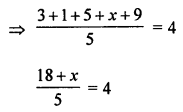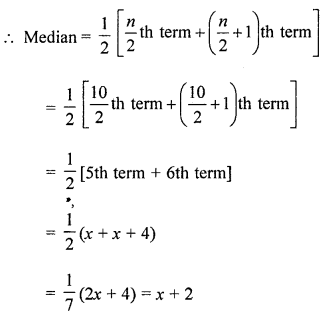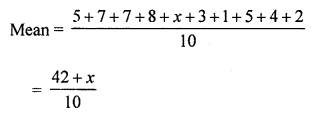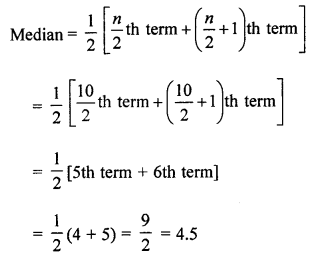## ML Aggarwal Class 7 Solutions for ICSE Maths Chapter 17 Data Handling Objective Type Questions

Mental Maths

Question 1.
Fill in the blanks:
(i) The number of times a particular observation occurs in a data is called ………. of that observation.
(ii) The frequency of 9 is written symbolically as ………. using tally marks.
(iii) In a bar graph, the height (or length) of a bar is equal to or proportional to the frequency of the ……….
(iv) Double bar graphs help us in comparing two ……….. at a glance.
(v) The most common representative value of a statistical data is the ……….. of the data.
(vi) Mode of a data is the observation which occurs …………. number of times in the given data.
(vii) The arithmetic mean of first 10 natural numbers is ……….
(viii) When a die is rolled, the probability of getting a number more than 5 is ………..
(ix) The probability of an ……….. event is 0.
(x) The probability of a ………… event is 1.
(xi) Median is one of the observations is the data if the number of observations is ………..
Solution:
(i) The number of times a particular observation occurs
in a data is called frequency of that observation.
(ii) The frequency of 9 is written symbolically as IIII using tally marks.
(iii) In a bar graph, the height (or length) of a bar is equal to
or proportional to the frequency of the corresponding observation.
(iv) Double bar graphs help us in comparing two collection data at a glance.
(v) The most common representative value of
statistical data is the arithmetic mean of the data.
(vi) Mode of a data is the observation which occurs
a maximum number of times in the given data.
(vii) The arithmetic mean of first 10 natural numbers is 5.5.
(viii) When a die is rolled, the probability of getting
a number more than 5 is $$\frac { 1 }{ 6 }$$.
(ix) The probability of an impossible event is 0.
(x) The probability of a sure event is 1.
(xi) Median is one of the observations is the data
if the number of observations is odd.

Question 2.
State whether the following statements are true (T) or false (F):
(i) Mean is the value of observation that occurs most frequently.
(ii) The range of the data -3, 4, -5, 1, -2, 0 is -9.
(iii) Mode is always one of the numbers in a data.
(iv) There is only one mode for a given data.
(v) Mean is always one of the numbers in a data.
(vi) The data 6, 4, 3, 8,9, 12, 13, 9 has mean 9.
(vii) Median of the data may or may not be from given given data.
(viii) The measures of central tendency may not lie between the maximum and minimum value of data.
(ix) If the extreme observations on both ends of a data arranged in ascending order are removed, then the median gets affected.
(x) The probability of getting an ace out of a deck of playing cards can be greater than 1.
(xi) Median of 4, 5, 9, 2, 6, 8, 7 is 2.
Solution:
(i) Mean is the value of observation that occurs most frequently. (False)
Correct:
It is mode not mean.
(ii) The range of the data -3, 4, -5, 1, -2, 0 is -9. (False)
Correct:
Range is difference between 4 and -9 = 13 not 9.
(iii) Mode is always one of the numbers in a data. (True)
(iv) There is only one mode for a given data. (False)
Correct:
Mode can be more than one.
(v) Mean is always one of the numbers in a data. (False)
Correct:
It can be other than the given number.
(vi) The data 6, 4, 3, 8, 9, 12, 13, 9 has mean 9. (False)
Correct:(vii) Median of the data may or may not be from given given data. (True)
(viii) The measures of central tendency may not lie between
the maximum and minimum value of data. (False)
Correct:
It lies between minimum and maximum value of the data given.
(ix) If the extreme observations on both ends of a data arranged
in ascending order are removed, then the median gets affected. (False)
Correct:
There is no effect on median.
(x) The probability of getting an ace out of a deck of playing cards
can be greater than 1. (False)
Correct:
Probability occurs between 0 to 1 not greater than 1.
(xi) Median of 4, 5, 9, 2, 6, 8, 7 is 2. (False)
Correct:
Median of (2,4, 5, 6, 7, 8, 9) is the 4th term which is 6.

Multiple Choice Questions

Choose the correct answer from the given four options (3 to 18):
Question 3.
Which of the following is not a central tendency of a data?
(a) Mean
(b) Median
(c) Mode
(d) Range
Solution:
Range is not a central tendency of a data. (d)

Question 4.
The difference between the highest and the lowest observations in a data is its
(a) frequency
(b) width
(c) range
(d) mode
Solution:
The difference between the highest and the lowest
observations in data is its range. (c)

Question 5.
A cricketer scored 38, 79, 25, 52, 0, 8, 100 runs in seven innings. The range of the runs scored is
(a) 100
(b) 92
(c) 52
(d) 38
Solution:
Range = Difference of the highest and lowest observation
Range is 100 – 0 = 100 (a)

Question 6.
If the mean of 3, 1, 5, x and 9 is 4, then the value of x is
(a) 6
(b) 4
(c) 2
(d) 0
Solution:
Mean of 3, 1, 5, x, 9 is 418 + x = 20
⇒ x = 20 – 18 = 2 (c)

Question 7.
The number of goals scored by a football team in a series of matches are: 3, 5, 0, 1, 2, 0, 4, 1, 3. The median of this data is
(a) 3
(b) 2
(c) 2.5
(d) 1
Solution:
Goals in the matches are:
3, 5, 0, 1, 2, 0, 4, 1, 3
Arranging in order: 0, 0, 1, 1, 2, 3, 4, 5
Which are 9 which is odd
median = $$\frac { 9+1 }{ 2 }$$ = 5th term = 2 (b)

Question 8.
The median of the first 49 natural numbers is
(a) 24
(b) 25
(c) 25.5
(d) 26
Solution:
Median of first 49 natural number (i.e., 1 to 99)
= $$\frac { 49+1 }{ 2 }$$ = 25th term = 25 (b)

Question 9.
The mean of three different natural numbers is 40. If lowest is 19, what could be highest possible number of remaining two numbers?
(a) 40
(b) 71
(c) 81
(d) 100
Solution:
The mean of three different natural numbers = 40
Lowest = 19, then highest possible of the remaining two numbers
Total = 40 × 3 = 120
Lowest = 19
Remaining total = 120 – 19 = 101
Both of the remaining numbers are greater than 19
So, if second is 20, then third = 101 – 20 = 81
Highest = number = 81 (c)

Question 10.
The mode of the data:
3, 5, 1, 2, 0, 2, 3, 5, 0, 2, 1, 6 is
(a) 6
(b) 3
(c) 2
(d) 1
Solution:
Mode of 3, 5, 1, 2, 0, 2, 3, 5, 0, 2, 1, 6 = 2
as it came highest times (c)

Question 11.
The probability of a sure event is
(a) 0
(b) $$\frac { 1 }{ 4 }$$
(c) $$\frac { 1 }{ 2 }$$
(d) 1
Solution:
The probability of a sure event is 1. (d)

Question 12.
The probability of an impossible event is
(a) -1
(b) 0
(c) $$\frac { 1 }{ 2 }$$
(d) 1
Solution:
The probability of an impossible event is 0. (b)

Question 13.
The probability of a newly born baby to be a girl is
(a) 0
(b) $$\frac { 1 }{ 4 }$$
(c) $$\frac { 1 }{ 2 }$$
(d) 1
Solution:
The probability of a newly bom baby to be a girl is $$\frac { 1 }{ 2 }$$. (c)

Question 14.
The probability of selecting letter G from the word GIRL is
(a) 1
(b) $$\frac { 1 }{ 2 }$$
(c) $$\frac { 1 }{ 4 }$$
(d) $$\frac { 1 }{ 3 }$$
Solution:
Probability of letter G from the word GIRL is $$\frac { 1 }{ 4 }$$. (c)

Question 15.
The probability of selecting a vowel from the word ALPHABET is
(a) $$\frac { 1 }{ 2 }$$
(b) $$\frac { 1 }{ 7 }$$
(c) $$\frac { 2 }{ 7 }$$
(d) $$\frac { 3 }{ 8 }$$
Solution:
The probability of selecting a vowel
from the word ALPHABET (Vowels are A, A, E)
= $$\frac { 3 }{ 8 }$$ (d)

Question 16.
When a die is thrown, the probability of getting a composite number is
(a) $$\frac { 1 }{ 2 }$$
(b) $$\frac { 1 }{ 3 }$$
(c) $$\frac { 1 }{ 4 }$$
(d) $$\frac { 2 }{ 3 }$$
Solution:
When a die is thrown,
probability of a composite number (4, 6) = $$\frac { 2 }{ 6 }$$ = $$\frac { 1 }{ 3 }$$ (b)

Question 17.
A bag contains 5 white balls and 10 black balls. The probability of drawing a white ball from the bag is
(a) $$\frac { 5 }{ 10 }$$
(b) $$\frac { 5 }{ 15 }$$
(c) $$\frac { 10 }{ 15 }$$
(d) 1
Solution:
A bag contains 5 white and 10 black balls.
Total balls in the bag = 5 + 10 = 15
Probability of drawing a white ball = $$\frac { 5 }{ 15 }$$ = $$\frac { 1 }{ 3 }$$ (b)

Question 18.
In a school, only 2 out of 5 students can participate in a quiz. What is the probability that a student picked at random makes it to the competition?
(a) 50%
(b) 40%
(b) 30%
(d) 20%
Solution:
In a school, 2 out of 5 students can participate in a quiz
Probability of 2 students picked at random = $$\frac { 2 }{ 5 }$$
= $$\frac { 2 }{ 5 }$$ × 100 = 40% (b)

Value Based Questions

Question 1.
The following table shows the time (in hours) spent by a student of class VII in a day:

 Activity School Sleeping Playing Watching T.V. Studying Others Number of Hours 8 8 1 3 2 2

Draw a bar graph to represent the above data. Is it correct to watch T.V. for 3 hours and studying just 2 hours daily?
Solution:
Bar graph of the given data is given below:Watch T.V. for 3 hours and studying only for 2 hours is not correct.
A student must give more time to study.

Question 2.
In a game, a fair coin is tossed. A person is paid ₹ 5 if he gets head and he pays ₹ 2 if he gets tail. Find the probability of winning ₹ 5. Is gambling a good way of earning money?
Solution:
In a game, a fair coin is tossed.
A person gets ₹ 5 for head and he pays ₹ 2 for getting tail.
So, probability of winning ₹ 5 = $$\frac { 1 }{ 2 }$$
It ruins the whole family.
At the end, he loses everything.

Higher Order Thinking Skills (HOTS)

Question 1.
The mean of 6 observations is 17.5. If five of them are 14, 9, 23, 25 and 10, find the sixth observation.
Solution:
Mean of 6 observations = 17.5
Total = 17.5 × 6 = 105
Sum of 5 observations = 14 + 9 + 23 + 25 + 10 = 81
6th observation = 105 – 81 = 24

Question 2.
The mean height of 10 students is 151.8 cm. Two more students of heights 157.6 cm and 154.4 cm join the group. What is the new mean height?
Solution:
Mean height of 10 students = 151.8 cm
Their total height = 151.8 × 10 = 1518 cm
Heights of two more students = 157.6 + 154.4 = 3120
Sum of 10 + 2 = 12 students = 1518 + 312 = 1830 cm
Their mean height = $$\frac { 1830 }{ 12 }$$ = 152.5 cm

Question 3.
The following observations have been arranged in ascending order. If the median of the data is 13, find the value of x:
3, 6, 7, 10, x, x + 4, 19, 20, 25, 28
Solution:
Following observations have been arranged in order:
3, 6, 7, 10, x, x + 4, 19, 20, 25, 28
Here, n= 10 which is evenBut median = 13
x + 2 = 13 ⇒ x = 13 – 2 = 11
x = 11

Question 4.
If the mean of the data (given below) is 4.5, then find the median of the data:
5, 7, 7, 8, x, 3, 1, 5, 4, 2
Solution:
Mean of following data = 4.5
5, 7, 7, 8, x, 3, 1, 5, 4, 2
Here n = 10$$\frac { 42+x }{ 10 }$$ = 4.5
⇒ 42 + x = 4.5 × 10
⇒ 42 + x = 45
⇒ x = 45 – 42 = 3
Now arranging in ascending order,
1, 2, 3, 3, 4, 5, 5, 7, 7, 8Question 5.
Find the probability of getting 53 Sunday’s in a leap year.
Solution:
Probability of 53 Sunday’s in a leap year
Number of weeks in 366 days = 52
and remaining days = 2
Number of days in a week = 7
Probability = $$\frac { 2 }{ 7 }$$